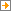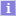Szkoła Główna Handlowa w Warszawie - Centralny System Uwierzytelniania Nie jesteś zalogowany | zaloguj się

# Mathematics of Finance121191-D Wykład (WYK) Semestr letni 2019/20

## Informacje o zajęciach (wspólne dla wszystkich grup)

 Liczba godzin: 30 Limit miejsc: (brak limitu) Zaliczenie: Egzamin Zakres tematów: Simple interest. Simple discount. The equivalence of simple interest rates. Time measurement in finance Bank discount (interest in advance). Equivalence of interest rate and discount rate. Commercial bills - discounting, equivalence, portfolio. Treasury bills - primary market auction, discount rate, equivalent rate of return. Compound interest: periodic compounding, continuous compounding. Annual accumulation factor. Effective rate of interest. Nominal rate of interest. Equivalent rates of interest. Compound discounting. Varying interest rates, average rate. Inflation, deflation. Fischer's formula, real rate of interest. Time value of money. Exponential compounding. The value of dated amounts at different points in time: the present value (PV), the future value (FV). Equivalence of dated values, equation of value. Annuity. The main types of annuities. The present (PV) and the future value (FV) of annuity. Level payment annuity. Annuity accumulation factor, annuity discount factor. Valuation of annuities in Excel. Deferred annuity. Perpetuity. General annuity. Assessment of financial investments: net present value (NPV), internal rate of return (IRR). Mathematical aspects of IRR existence and calulation. Discounted payback period, duration. Assessment of investments in Excel. Methods of loan amortization. The general principle of loan repayment. Retrospective, and prospective approach. Amortization table. Level payments, level principal repayments. Loan repayment under simple interest. The annual percentage rate of charge for consumer credits (APR). Ambiguity of APR under simpe interest. Use of Excel.

## Grupy zajęciowe

zobacz na planie zajęćGrupa Termin(y) Prowadzący MiejscaAkcje
Opisy przedmiotów w USOS i USOSweb są chronione prawem autorskim.
Właścicielem praw autorskich jest Szkoła Główna Handlowa w Warszawie.